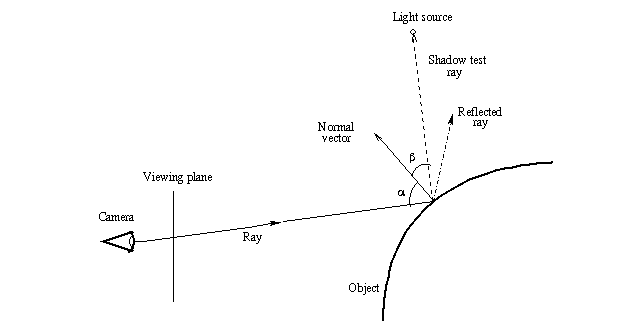POV-Ray : Documentation : 1.3.10.3 Short introduction to raytracingPOV-Ray 3.6 Documentation Online View#### 1.3.10.3 Short introduction to raytracing

Before we start looking at the code, let's look briefly how raytracing works. This will help you understand what the script is doing.

The basic idea of raytracing is to "shoot" rays from the camera towards the scene and see what does the ray hit. If the ray hits the surface of an object then lighting calculations are performed in order to get the color of the surface at that place.

The following image shows this graphically:First a ray is "shot" in a specified direction to see if there is something there. As this is solved mathematically, we need to know the mathematical representation of the ray and the objects in the scene so that we can calculate where does the ray intersect the objects. Once we get all the intersection points, we choose the closest one.

After this we have to calculate the lighting (ie. the illumination) of the object at the intersection point. In the most basic lighting model (as the one used in the script) there are three main things that affect the lighting of the surface:

• The shadow test ray, which determines whether a light source illuminates the intersection point or not.
• The normal vector, which is a vector perpendicular (ie. at 90 degrees) to the object surface at the intersection point. It determines the diffuse component of the lighting as well as the direction of the reflected ray (in conjunction with the incoming ray; that is, the angle alpha determines the direction of the reflected ray).
• The reflected ray, which determines the specular component of the lighting and of course the color of the reflection (if the object is reflective).

Do not worry if these things sound a bit confusing. Full details of all these things will be given through this tutorial, as we look what does the raytracing script do. The most important thing at this stage is to understand how the basic raytracing algorithm works at theoretical level (the image above should say most of it).

Let's just look at the raytracer source code line by line and look what does it do